## Sunday, November 30, 2008

### A Teaching Statement

Such as it is, I have prepared a reflective (use caution in direct sunlight) teaching statement. I'm neither a deep thinker, nor a proficient writer, so I can't convince myself that the result is any good. Either way, here's what I came up with:

I have no idea why I bother teaching.

I can convince myself that many of my students will need to know mathematics in their future careers. My science students clearly need mathematics, but so do the business students. The fine arts are a little harder to justify, so perhaps pawn it off on "general education." Numeracy, people claim, is practically as important as literacy, even if it doesn't get the same attention (in fact, some people pride themselves on their innumeracy). If nothing else, mathematics courses are a requirement at the University, and I have enough of an understanding of the subject to try to get others through it.

So my issue with teaching isn't that the content isn't important.

My issue with teaching is that, in this connected age, there are effectively unlimited sources for the knowledge that I am supposed to cover in a calculus class. Certainly there has always been the textbook, and I love for my students to read it. But now, online, there are more places to learn calculus than one could possibly use. Entire lectures and individual problems and short snippets get posted as videos to YouTube. Wikipedia contains more information than any one person can know (and it's constantly growing!). I also must compete against the Massachusetts Institute of Technology, who not long ago started posting all course materials online (for free, accessible by anybody).

So why would a student bother coming to my class? Anything I'll be covering in class could be found online, from the relative comfort of a dorm room. It's not my job to tell students when to think about calculus, so I have no attendance policy. Who am I to tell them when they should be learning? If they are motivated enough to learn the material on their own, I don't want to frustrate their ambitions by making them come to class.

It is this sense of competition that drives my lectures. When planning lectures, I make sure to use examples that aren't covered in the book. I also try to find as many "fun" examples and extra problems as possible. Students don't necessarily want to look at other resources to learn the material I will be teaching. Even motivated students might not take that step. Students might not know where to look on Wikipedia for interesting mathematics that is, at the same time, easy enough to digest without having taken lots of math classes. It's my job to show these things to my students.

It's lucky, then, that mathematics is so fascinating. Surprising amounts can be boiled down and understood without having spent years taking math classes and reading math textbooks. This is my job. Anybody can present material, and cover examples. I think it is important to go further, to find more interesting examples, and historical tidbits. I always hope to show my students some of the beautiful things in mathematics.

During the times I am doing fun examples and presenting material that isn't strictly necessary for the class it is easy to be an excited speaker. However, there is something interesting to be found in nearly every example, and I try to keep my enthusiasm high throughout every lecture. Judging from course feedback, my students feed off this energy, and are more motivated to learn the material. If I can create motivated learners, who start seeing interesting things in the content I teach, then all of those external resources are more likely to be accessed and dug through. All the material in world is worth nothing if students aren't interested in looking at it. It is my hope that I can encourage some to embrace this interest.

## Thursday, November 27, 2008

### Slackware!

I've been using Ubuntu for a while. It's got some great features, notably it's package management and update setup (which my friend has complained about, but I've always appreciated). But I've recently been frustrated with things responding slowly on my computer. I've tried turning off services and such, but it never seemed to improve. In the past, I've used gentoo and slackware, and I remembered them being a bit snappier for me. With a new version of slackware out, I thought it was about time to try something new(ish).

So I downloaded the iso images and burned them to cd. I backed up some data (been meaning to look at Amazon's S3 online storage... apparently that'll be another day though), and took the plunge. The setup seemed to be going well, until a failure when it got to installing mysql. Retry, and still fail. Took the disc out, tried cleaning it off, and still fail. So I came back to my ubuntu install, and found these directions about installing slackware without cds. I only skimmed through them, but got a good enough idea how things should go. Since the cds I had would properly boot and let me run setup, I think most of those directions weren't too necessary anyway. I downloaded new iso images, and rebooted with my install cd in the drive. When setup came around to asking for my source, I pointed it at the mount point (which I had made, and mounted things to before running setup) for my iso image, and was good to go.

One thing that confused me was that there are multiple install cds, but the directions at the link above didn't seem to care about that. They only indicated one disk. I mounted discs one and two in different locations, but I think you could save a step and mount both in the same place before running setup. I would have also mounted disc three, but it gave me an error about being able to find loop devices (perhaps I'm only allowed 2?). Anyway, after disc one was done, I was still in setup, and told it to install more packages from the second mount point. Seemed to go fine.

After a reboot, there was lilo, waiting for me. My ubuntu image wasn't there, but I've edited /etc/lilo.conf before, so I wasn't too concerned. First step: make a non-superuser - useradd. Next up, start the windowing environment (as pretty as the command line is) - startx. FAIL. "could not open default font 'fixed'", then "waiting for X server to begin accepting connections" and "giving up". That's not what I wanted to see.

Lynx, to google, and asked about it. This turned up, and using alt-F2 and alt-F1 to bounce between terminals, I got the commands entered. Fingers crossed, 'startx'... Hurray! Xfce.

Now I get to configure things again. Always a good time. I've got firefox 3 installed, and twitterfox, and the flash plugin, and my old bookmarks. I've got my desktop set back up basically the way I had it. That's probably enough for one day. Besides XEmacs and a terminal, that's pretty much all I use anyway. On that note, the default terminal isn't behaving at all for me. I type, and it doesn't even show the characters I type. I've been temporarily using xterm, but it's something I'll have to look at some more.

## Wednesday, November 26, 2008

### 72 Pencils

I've been wanting to make my own copy of the 72 pencil sculpture by George W. Hart since I first saw it online. Have a look at it here, and explore his other works - they're awesome. I've had two boxes of 48 pencils sitting in my closet for a few years now, waiting for the day I was ready to make this thing. Yesterday I finally decided to make it, and went to Michael's to buy some styrofoam. I spent... probably 5 hours yesterday trying to work it out, and didn't get it. I woke up this morning, and stared at it for another 4 hours or so. Finally, I started over, and within about another hour I had it. Turns out, all of my first attempts had been started wrong. Idiot.

After I got it together:I thought I'd see what happened when I removed the rubber bands. Perhaps I removed them without enough care, but it ends up like this:So I started over, taking pictures as I went. If you want to make your own, it really isn't that bad. I just wasn't being careful enough for my first... 10 tries or so :) What'll you need? 72 pencils (4 hexagonal cylinders, 18 pencils each) of course. I used a bit of styrofoam, a 1 inch think circular disc with diameter ~6 inches. To hold things together, I also used 4 pieces of string (each about a foot long, I'd guess) and 6 rubber bands. My cats wanted to help too: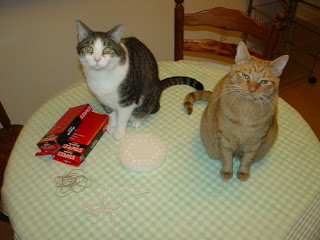I arranged the first 18 pencils in a cylinder poking into the styrofoam. The hexagon was bigger than it needed to be, and the pencils were wobbly, and not equally spaced. You might also noticethat I put one rubber band around the styrofoam, which I did before any pencils were in. Next, start weaving some pencils into the uprights. You may find the original picture helpful. Here's a picture of the early stage of the process, for me: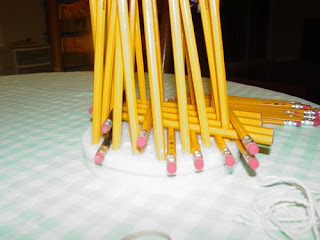I have a couple of extra pencils (the black ones) in there for balance. They'll get removed later.

Keep stacking these pencils up, until it looks something likeAfter that, I tied a loop of string around each end of both horizontal cylinders. This permitted me to then put a rubber band around each end of the horizontal cylinders (and remove the strings)Now push the rubber band off the styrofoam base, to hold the bottom of the vertical cylinder. Put a rubber band around the top of the vertical cylinder, flip the whole thing over (so the styrofoam is up in the air), and pull the styrofoam off (which I found surprisingly easy). Now you've just got the forth cylinder to get in there. I had to follow the original picture, and carefully put pencils where they should be according to the picture. After the first few, it's pretty easy to see where they should go.

So, I don't know how helpful that was. It's really not too hard, as long as you start things correctly. This was my problem the first several times. But it all worked out in the end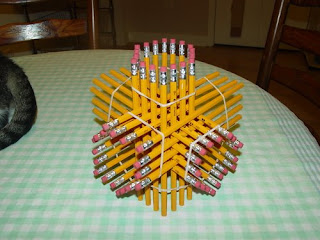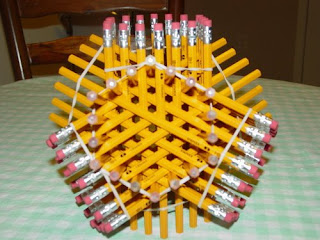Of course, there's still those rubber bands. I'm not entirely sure how to get rid of them, so they'll probably stay for a while.

Update 20081201: Ok, with a little super glue one some obviously loose penciles, I was able to remove the rubber bands. Hoping it stays together for a while now.

### Happy Thanksgiving

Yeah, yeah. I know I'm a day early. That's just how I'm making my Thanksgiving break work out. Anyway, I did some cooking, and thought I'd share.

First up, spiced fruit: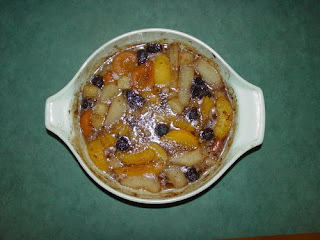It's a pretty easy recipe. Basically all you need is some canned fruit, some spices, and some butter. I used peaches, pears, pineapples, apricot halves, and black cherries. Empty the entire can (juice and all) of pineapples, and drained can of apricot halves and black cherries into a large oven-safe dish. Drain the juice from the peaches and pears into a separate dish, and put the fruit in the oven-safe dish. Use as much of the peach/pear juice as you'd like to fill up the main dish, and discard (or find some other way to use) the remaining juice. Next, add 1tsp cinnamon, and 1/2tsp nutmeg (my mom also uses 1tsp curry powder). Slice 1/2 stick of butter into pieces and set on top of the dish. Cook at 350 for 1 hour.

Next up, apple and cranberry chicken skillet dish:This is basically the recipe here, with approx 5oz (I had a 10oz package, and used half) of dried cranberries instead of the onion.

Finally, pumpkin apple streusel cake: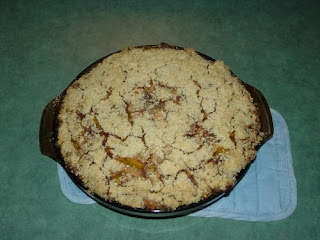with some vanilla ice cream:following the recipe at epicurious.com.

I made some crescent rolls too, and will make some stuffing (with sausage!), and hot cider at some point. But these were the main dishes. OM NOM NOM.

Hope everybody has a nice Thanksgiving, and your cooking goes as well (better, actually - I gave myself a bit of a cut) as mine. Oh, and travelling, too - I'm happy I got to avoid that part.

## Saturday, November 22, 2008

### Course Evaluations

With the end of the semester approaching, we've got course evaluations coming up. While the list of questions that every class gets its pretty decent, I like to add some more personalized questions to my evaluations. Currently what I've got is:
1. What feedback can you offer about the webwork system? Are there changes you would like to see, concerning the interface or features? How could it be made more useful for you?
2. How did you feel about the combination of webwork and written homework? Did you feel they were fairly balanced?
3. What parts of our course Collab page did you use, besides homework assignments? Did you look at homework or exam solutions? Did you follow any of the additional links under resources? In what ways do you wish Collab had been used differently?
4. Were there certain topics or sections you felt were taught particularly well or particularly poorly?
5. Is there anything you wish you would have been told about the class, before it started? What advice would you give to a future student of this course?
6. What changes do you think Nick could make to improve this course?
I guess for these first few questions to make sense, you might like to know a few things about my course. In addition to written homework, consisting of problems from the textbook, I also use WebWork, which is an online math homework system. I pick collections of problems every week that the students complete online. This cuts down on grading, but still gives the students a chance to do problems for points. Collab is UVAs course management... system, it's 'Powered by Sakai'. I post homeworks, old exams, exam solutions, and some miscellaneous links there.

My questions for you: What do you look for in a course evaluation? What questions return the most meaningful answers for you, as an instructor? What questions, as a student, do you wish were asked?

Update 20081129:
I thought up another question: Did you use any external sources to help you in this class? For example, books besides the textbook, or any websites? If so, what did you use, and to what extent?

## Monday, November 17, 2008

### I Can Has Thesis?

No, I can't. Not for a while. Because I can't seem to figure out the things I'm supposed to be looking at, and because I distract myself by other things. One of the other distractions, recently, has been playing around with polynomials. If you've been following me here recently, you've seen this already. What you haven't seen is what's happened since then.

I decided to try out WordPress, because I knew it had good built-in support for LaTeX, which Blogger doesn't have. So, over at sumidiot.wordpress.com, I've been keeping a more regular log of the work I've been doing, which in the past few days has only been messing about with quartic and quadratic polynomials. And I think I'm about done with them. I have determined when a quartic can be decomposed, and what it's decompositions are. The final result seems to be that it is the composition of two quadratics iff it is symmetric around a vertical line. I won't redo all the equations and things, because they're already at the above pages.

So... I know that's not thesis quality work. It's probably something people have done before, and it took well under a week to work out. All the same, it's been the most independently motivated I've been by working on a math question since playing with FLT in high school (yes, I really did - and no (clearly) I didn't get anywhere). I've had a great time playing with this question, and I've still got more I'd like to look at. In the mean time, I really ought to be thinking about my actual research, which is, sadly, entirely unrelated.

I've also been trying to decide what to do with that wordpress account. The subtitle I've currently got up there indicates it might be a fork of this blog, for the mathematical content. I've debated about trying to use it regularly to just write up whatever I've been looking at - for research or for fun. Writing things up is good practice, and helps organize your thoughts. Both of these would probably be good for me. Do I drop this blogspot page? Or drop the wordpress one? Post weekly wrapup links from here to the wordpress page? Post wrapup links when some sort of progress has been made? I guess time will tell. I know you all can't wait to see what happens. I'll keep you posted. If Blogger would just natively support LaTeX...

## Wednesday, November 12, 2008

I've been playing with polynomials a bit recently, and so I expect this post will be the first of several, explaining my explorations. The question I'd like to look at today is: When is a quartic (degree 4) polynomial the composite of two quadratics (degree 2)? Can every quartic be written as such a composite? Uniquely?

I wouldn't be surprised to find somebody has written this up before. In fact, they've probably dealt with more general questions, in an easier manner. All the same, this is how I spent my day, so I thought I'd share.

You might notice that 4 is the smallest degree polynomial that can be written as a composite of lower degree polynomials. If $p_i$ is a polynomial of degree $d_i$ (for $i=1,2$), then $p_1\circ p_2$ has degree $d_1\cdot d_2$. So if we wanted a cubic as a composite of lower degree polynomials, we'd have trouble doing it, and similarly for quadratics. However, these same considerations help us out a little bit. Suppose $f$ is a quartic that can be written as the composite $p_1\circ p_2$ of quadratics, and that T is a (non-constant) linear function. Then $T\circ f=T\circ p_1\circ p_2=(T\circ p_1)\circ p_2$, showing that $T\circ f$ is still the composite of two quadratics. The same argument works in reverse: if $T\circ f=p_1\circ p_2$, then $f=T^{-1}\circ p_1\circ p_2$ (and $T^{-1}$ exists, since $T$ was assumed linear), which is still the composite of quadratics.

What's the point of this linear function $T$? Well, suppose
$f=ax^4+bx^3+cx^2+dx+e.$
Let $T=\frac{1}{a}(x-e)$, and then notice that $T\circ f$ is monic (leading coefficient 1) and has 0 constant term. If the polynomial $T\circ f$ can be written as a composition of quadratics, then by the previous paragraph, $f$ can be written similarly. So, we can make our lives easier, and assume that $a=1$ and $e=0$.

Let $p_1=a_1x^2+b_1x+c_1$ and $p_2=a_2x^2+b_2x+c_2$. We'd like to know if we can write our $f$ above as $p_1\circ p_2$. If you're following along at home, I'll wait while you write out the composite $p_1\circ p_2$. To check your work (and mine), when you want $f=p_1\circ p_2$, you set the corresponding coefficients equal, and obtain the following system of equations:
$a=a_1a_2^2$
$b=2a_1a_2b_2$
$c=2a_1a_2c_2+a_1b_2^2+a_2b_1$
$d=2a_1b_2c_2+b_1b_2$
$e=a_1c_2^2+b_1c_2+c_1$

Our simplifications let us write $a=1$ and $e=0$. Let's also go ahead and assume $a_1=a_2=1$ (that is, our quadratics are monic), to make our calculations easier. It's also believable (I hope) that we haven't lost anything with this assumption, but perhaps I should think about this some more. I had convinced myself of it in the office this afternoon. Anyway, this makes our equations
$b=2b_2$
$c=2c_2+b_2^2+b_1$
$d=2b_2c_2+b_1b_2$
$0=c_2^2+b_1c_2+c_1$

Remember that we're assuming $b$, $c$, and $d$ were fixed, and are hoping to find $b_1,b_2,c_1$, and $c_2$. The first line clearly makes it easy to find $b_2=b/2$, and we can substitute that in the remaining lines:
$c=2c_2+b_1+(b^2/4)$, or $c-b^2/4=2c_2+b_1$
$d=(b/2)(2c_2+b_1)$, or $2d/b=2c_2+b_1$
$0=c_2^2+b_1c_2+c_1$

Now we're making progress. But first, we've made an assumption in the line $2d/b=2c_2+b_1$. What is it? Well, to divide by $b$, we assume $b\neq 0$. If, alternatively, $b=0$, then also $d=0$ (since $d$ is a multiple of $b$) and $b_2=0$. With all of these values being 0, the above system has 2 equations (the first and third) and 3 unknowns ($b_1$, $c_1$, and $c_2$). We can solve for $b_1$ and then $c_1$ in terms of a free variable $c_2$. Thus we obtain
Proposition: A quartic $x^4+cx^2+dx$ with $d\neq 0$ can not be written as the composition of two quadratics. The quartic $x^4+cx^2$ can be written as the composite $p_1\circ p_2$ where
$p_1=x^2+(c-2c_2)x+(c_2^2-cc_2)$
$p_2=x^2+c_2$
and $c_2$ can be any value.

Ok, so, assuming $b\neq 0$, what happens? The equations
$c-b^2/4=2c_2+b_1$
$2d/b=2c_2+b_1$
mean that $c-b^2/4=2d/b$, which we chose to re-write as $8d=4bc-b^3$. This is a requirement on the coefficients of the original quartic. However, if this is satisfied, then we can pick
$b_1=2d/b-2c_2$
$c_1=c_2^2-2dc_2/b$
in terms of a free variable $c_2$. We conclude by stating the final proposition, which can be checked by brute force (like everything else above):
Proposition: If $b\neq 0$ in the quartic $f=x^4+bx^3+cx^2+dx$, then $f$ can be written as the composite of quadratics only if $8d=4bc-b^3$ - in which case $f=p_1\circ p_2$ for
$p_1=x^2+(2d/b-2c_2)x+(c_2^2-2dc_2/b)$
$p_2=x^2+b/2 x+c_2$
where $c_2$ can be any value.

So there you have it. Not all quartics can be written as composites of quadratics. For those that can, there are a 1-parameter family of choices for monic quadratic factorizations.

Update 20081115: I've been thinking about my reduction that made the quadratics monic. I've been trying to decide if it was necessary. Playing around with the equations some more, it's not too hard to tell that you can't get rid of the relation $8d=4bc-b^3$ on the coefficients of the quartic, even if you don't assume your quadratics are monic. If you allow non-monic quadratics (but still want the composition to be monic), you have a free choice of value for $a_1$ (as long as it's positive) and for $c_2$ still, and the other coefficients can be solved for in terms of these two values (and the coefficients for the quartic).

Also, the two propositions could be combined. The relation $8d=4bc-b^3$ is present in both propositions, and there's no reason to assume $b\neq 0$ in that relation. Of course, we do end up dividing by $b$ to solve for coefficients in $p_1$ and $p_2$, so it's something to keep in mind.

Ok, I better go do some real work. I've still got other polynomial questions to look at, but they'll have to wait.

## Sunday, November 9, 2008

### A Fun Category

During my time in grad school, I have often wondered what I would talk about if I were invited to give a talk to an undergraduate audience. Like the math club here, or where I went for undergrad. I've generally had a good time with categories, and am pretty convinced something like the following would work as a fun talk. Perhaps my definition of fun needs some work.

For starters, let's just think about sets for a while - and I'll have finite sets in mind, but mostly that shouldn't matter. If $S$ and $T$ are sets, the Cartesian product is the set $S\times T=\{(s,t)|s\in S,t\in T\}$. What makes this new set useful? Why should we care about it? I suppose it depends on who you ask, but since I've got categories in mind, I want to think about functions (=maps) between sets. The product comes with 2 maps, I'll call them $\pi_S$ and $\pi_T$. The map $\pi_S:S\times T\to S$ is defined by $(s,t)\mapsto s$, and the map $\pi_T$ is similar. Now, if I have a set $A$, and a map $f:A\to S\times T$, then I can compose $f$ with either $\pi_S$ or $\pi_T$ and obtain maps $\pi_S\circ f:A\to S$ and $\pi_T\circ f:A\to T$. That is, I can tease out the component pieces of the map $f$. On the other hand, if I started with a set $B$, and maps $g:B\to S$ and $h:B\to T$, I can define a map $(g,h):B\to S\times T$ via $b\mapsto (g(b),h(b))$.

So when I think about the product of two sets, I notice that maps to the product are essentially the same as individual maps to the sets I started with. This is how I want to think about the product in other contexts. If I start with two things (objects in some category) $x$ and $y$, I want their product $x\times y$ to be another thing (object) with the property that whenever I have component maps (a map to $x$ and a map to $y$), then I get a map to the product. Additionally, my product will come with two maps, $\pi_x$ and $\pi_y$, which I think of as the projections, following the example above.

What I'd like to do now is pick a fun context (category) and figure out what the product is in that category. In a category I'm supposed to have some collection of objects, and some collection of arrows between objects (satisfying various properties). So the category of finite sets, that I used above, just had finite sets as objects and functions as arrows. My new category will have as objects the positive integers. There will be a single arrow from $n$ to $m$ precisely when $n$ divides $m$.

So what is the product of $n$ and $m$ in this category? For now, let me call it $p$. Remember that $p$ comes with maps to $n$ and $m$ - which is to say, $p$ divides $n$ and $m$. There's a word for that - $p$ is a common divisor of $n$ and $m$. Which common divisor? Well, suppose $a$ is some other divisor. That means $a$ divides $n$ and $m$, or, in arrow notation, $a\to n$ and $a\to m$. But part of being the product was that whenever I have maps to $n$ and $m$, I get a map to the product, $p$. So $a\to p$, or, in other words, $a$ divides $p$. So, we've found that if $a$ is any other divisor of $n$ and $m$, then since $p$ is the product, $a$ must divide $p$. That makes $p$ the greatest common divisor.

Wasn't that fun?

These things can be 'dualized', and what you get is supposed to be called the sum. 'Dualizing' means flipping all the arrows around. Let's go back to finite sets for a minute. The product $S\times T$ came with maps $S\times T\to S$ and $S\times T\to T$. The dual of this idea (call it the coproduct, or sum), is an object, which I'll denote $S\coprod T$ and it has arrows $S\to S\coprod T$ and $T\to S\coprod T$. Notice how the arrows are going the 'other way' than they did for the product? For the product we mapped from the product to the components, whereas for the sum we map from the components to the sum. The sum also has the property that if $S\to A$ and $T\to A$, then $S\coprod T\to A$ (again, notice how this is flipped from the product case, where $A\to S$ and $A\to T$ gave $A\to S\times T$). The object, in sets, that is the coproduct is more commonly known as the disjoint union.

Exercise for the reader: what is the coproduct in the other category above, the 'divides' category?

You might ask why I decided that what was important, back in the beginning, was functions (maps). It's a good question. I've read somewhere (though I forget where) that thinking about objects by themselves is ok, but thinking about how they related to other objects (via maps) is even better. For a while I wasn't really sure why this should be, but I'm starting to put it together. If you go back and think about set cardinalities, you'll quickly notice that you are really asking about maps between sets, as a way to compare their sizes. If there is an injective or surjective map, you know one of the objects is at least as big as the other. If you've got a bijection, the two sets are the same size. It's hard to think about the size of a set, once you get past finite sets (but even there, really), without comparing it to other sets. And the way you compare sets is via maps between them. For an somewhat more advanced example, algebraic topology is wholly dedicated to maps between spaces. If you've got some space $X$, you decide that you'd really like to know about maps from various dimensional spheres into your space $X$. But perhaps that's a subject for another day.

## Sunday, November 2, 2008

### Fun with Series

The 'Sequences and Series' chapter of Calc 2 is the one I have been looking forward to the most, because there are some many awesome external diversions one can bring in to the class. I've already talked here about the first batch of fun things I mentioned in class, and thought I might update on some more things I've said, and plan on saying.

Now that we've talked about geometric series, it's possible to compute the area of the Koch snowflake, whose length we already computed in class when we talked about sequences. Our text (Stewart) has an exercise about the Cantor set, and the Sierpinski Carpet. The students are asked to compute the total length removed from [0,1] in order to make the Cantor set, and similarly the area removed to make Sierpinski's Carpet. I hope they found it surprising that the bits removed had the same length as the interval, and area as the square, even though there are still (clearly) infinitely many points remaining. While I was at it, I mentioned the Menger Sponge origami projects (and looking them up again convinced me that I should probably do one someday).

Though I've not yet figured out how, exactly, to bring it up in class, I think we're going to have a day and talk about cardinalities a little. I should at least show them that there is more than one infinity. Clearly it ties in to the examples from the previous paragraph, but it's also just good for their general education. Plus it's hugely fascinating. And they've spent all this time thinking about functions on the real line, they might as well know a little more about the line itself.

Even though I don't know a whole lot about it, since we were talking about p-series the other day I figured I should mention Riemann's ζ function, the associated Hypothesis, and the million dollar reward for a proof. They seemed to enjoy that there was a financial incentive, but at least one commented that there were surely easier ways to make a million dollars. I also showed how the divergence of the harmonic series implies that there are infinitely many primes, by considering the expression
$\sum_{n=1}^{\infty}\frac{1}{n}=\prod_{p}\frac{1}{1-p^{-1}}=\prod_{p}\left(\sum_{k=0}^{\infty} 1/p^k\right)$

These products are taken over all primes.

We also talked about the integral test in class, so I couldn't help bringing up the Euler-Mascheroni constant γ. I didn't say horribly much about it in class, but did mention how it can be used to approximate the number of terms in the harmonic series you would have to add up in order to get a chosen value. I worked through determining that it requires more than 12000 terms to just add up to 100.

The next thing I plan on talking about in class is sometimes referred to as the Kempner Series, and is obtained from the harmonic series by removing those terms whose denominators have some chosen digit. For example, remove all the terms that have a 0 as a digit in the denominator. What is initially surprising about this series is that it converges, though after some more thought you realize you've thrown out rather a lot of the series. Anyway, to show the Kempner series converges, you compare against a geometric series - all things we're talking about in my class.

Perhaps some of the most fun (besides, perhaps, the multiple infinities) will be once we get to Taylor series. I plan on presenting one of Euler's proofs that $\sum_{n=1}^{\infty}\frac{1}{n^2}=\frac{\pi^2}{6}$, by thinking about the Taylor series for $\sin(x)/x$. The way this works is to realize that $\sin(x)/x$ is zero at the non-zero integer multiples of $\pi$, so you think of the infinite polynomial that is the Taylor series as also being the product $\prod_{0\neq n}(1-x/(n\pi))$. After expanding this infinite product, you compare the coefficient of $x^2$ to the corresponding coefficient from the Taylor series, and Robert's your father's brother.

Another fun example I plan on doing (at least to some extent) is showing that
$\int_0^1 \frac{1}{x^x}dx=\sum_{n=1}^{\infty}\frac{1}{x^x}.$
This requires a bit of messing about, but it's so pretty that it's got to be worth it, right?

The final fun thing about Taylor series is it gives a nice segway into my research. Of course, I won't get too involved with telling them about my research, exactly. But I will mention that it's a direct analogy to Taylor series. And it'll give me a chance to talk about topology, which is always good.

Update 20081103: I realized I should include some text references here, sorry about that. The two books I had in mind were:
1. Gamma, by Julian Havil
2. Euler: The Master of Us All, by William Dunham